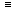Exam Mission

Exam Information| Govt Jobs.| Exam Preparation | Exam Study Material |Exam Date|Material PDF

1. Odd Days:
We are supposed to find the day of the week on a given date.
For this, we use the concept of 'odd days'.
In a given period, the number of days more than the complete weeks are called odd days.
2. Leap Year:
(i). Every year divisible by 4 is a leap year, if it is not a century.
(ii). Every 4th century is a leap year and no other century is a leap year.
Note: A leap year has 366 days.
Examples:
1. Each of the years 1948, 2004, 1676 etc. is a leap year.
2. Each of the years 400, 800, 1200, 1600, 2000 etc. is a leap year.
3. None of the years 2001, 2002, 2003, 2005, 1800, 2100 is a leap year.
3. Ordinary Year:
The year which is not a leap year is called an ordinary years. An ordinary year has 365 days.
4. Counting of Odd Days:
1. 1 ordinary year = 365 days = (52 weeks + 1 day.)1 ordinary year has 1 odd day.
2. 1 leap year = 366 days = (52 weeks + 2 days)1 leap year has 2 odd days.
3. 100 years = 76 ordinary years + 24 leap years
= (76 x 1 + 24 x 2) odd days = 124 odd days.
= (17 weeks + days)5 odd days.Number of odd days in 100 years = 5.
Number of odd days in 200 years = (5 x 2)3 odd days.
Number of odd days in 300 years = (5 x 3)1 odd day.
Number of odd days in 400 years = (5 x 4 + 1)0 odd day.
Similarly, each one of 800 years, 1200 years, 1600 years, 2000 years etc. has 0 odd days.
5. Day of the Week Related to Odd Days:
 No. of days: 0 1 2 3 4 5 6 Day: Sun. Mon. Tues. Wed. Thurs. Fri. Sat.